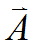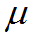﻿ Technical Notes > Applications in Electromagnetics > Magnetostatics > Divergence Form

Divergence Form

Navigation:  Technical Notes > Applications in Electromagnetics > Magnetostatics >

Divergence FormIn two dimensional geometry with a single nonzero component of, the gauge conditionis automatically satisfied.  Direct application of eq. (2.4) is therefore well posed, and we can proceed without further modification.

In 3D, however, direct implementation of eq. (2.4) does not impose a gauge condition, and is therefore ill-posed in many cases.  One way to address this problem is to convert the equation to divergence form using the vector identity
(2.6).

As long asis piecewise constant we can apply (2.6) together with the Coulomb gaugeto rewrite (2.4) as
(2.7)Ifis variable, we can generalize eq. (2.6) to the relation
(2.8)We assert without proof that there exists a gauge conditionwhich forces
(2.9).

The equations governingcan be stated asIt is not necessary to solve these equations; we show them merely to indicate thatembodies the commutation characteristics of the system.  The value ofis implied by the assertion (2.9).  Clearly, whenis constant, the equations reduce to, for whichis a solution.

Using the definition (2.9) we can again write the divergence form
(2.10).